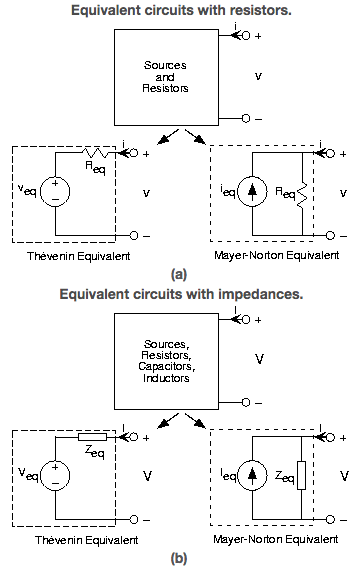# Equivalent Circuits: Impedances and Sources

When we have circuits with capacitors and/or inductors as well as resistors and sources, Thévenin and Mayer-Norton equivalent circuits can still be defined by using impedances and complex amplitudes for voltage and currents. For any circuit containing sources, resistors, capacitors, and inductors, the input-output relation for the complex amplitudes of the terminal voltage and current is

with

. Thus, we have Thévenin and Mayer-Norton equivalent circuits as shown in Figure 1.

#### Equivalent CircuitsFigure 1. Comparing the first, simpler, figure with the slightly more complicated second figure, we see two differences. First of all, more circuits (all those containing linear elements in fact) have equivalent circuits that contain equivalents. Secondly, the terminal and source variables are now complex amplitudes, which carries the implicit assumption that the voltages and currents are single complex exponentials, all having the same frequency.

#### Simple RC Circuit

Let's find the Thévenin and Mayer-Norton equivalent circuits for Figure 2. The open-circuit voltage and short-circuit current techniques still work, except we use impedances and complex amplitudes. The open-circuit voltage corresponds to the transfer function we have already found. When we short the terminals, the capacitor no longer has any effect on the circuit, and the short-circuit current

equals

. The equivalent impedance can be found by setting the source to zero, and finding the impedance using series and parallel combination rules. In our case, the resistor and capacitor are in parallel once the voltage source is removed (setting it to zero amounts to replacing it with a short-circuit). Thus,

. Consequently, we have

Again, we should check the units of our answer. Note in particular that

must be dimensionless. Is it?Swipe left and right to change pages.